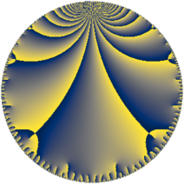# Properties

 Label 72.7.hLevel $72$ Weight $7$ Character orbit 72.h Rep. character $\chi_{72}(53,\cdot)$ Character field $\Q$ Dimension $24$ Newform subspaces $1$ Sturm bound $84$ Trace bound $0$

# Related objects

## Defining parameters

 Level: $$N$$ $$=$$ $$72 = 2^{3} \cdot 3^{2}$$ Weight: $$k$$ $$=$$ $$7$$ Character orbit: $$[\chi]$$ $$=$$ 72.h (of order $$2$$ and degree $$1$$) Character conductor: $$\operatorname{cond}(\chi)$$ $$=$$ $$24$$ Character field: $$\Q$$ Newform subspaces: $$1$$ Sturm bound: $$84$$ Trace bound: $$0$$

## Dimensions

The following table gives the dimensions of various subspaces of $$M_{7}(72, [\chi])$$.

Total New Old
Modular forms 76 24 52
Cusp forms 68 24 44
Eisenstein series 8 0 8

## Trace form

 $$24 q + 132 q^{4} + O(q^{10})$$ $$24 q + 132 q^{4} + 588 q^{10} + 4536 q^{16} + 11784 q^{22} + 75000 q^{25} + 25896 q^{28} + 122496 q^{31} + 74724 q^{34} - 56880 q^{40} - 74088 q^{46} + 197448 q^{49} - 149064 q^{52} - 232704 q^{55} - 196140 q^{58} - 1269024 q^{64} - 1585752 q^{70} + 514080 q^{73} + 327984 q^{76} - 1193088 q^{79} + 2813268 q^{82} - 3176832 q^{88} - 68856 q^{94} + 2960832 q^{97} + O(q^{100})$$

## Decomposition of $$S_{7}^{\mathrm{new}}(72, [\chi])$$ into newform subspaces

Label Dim $A$ Field CM Traces $q$-expansion
$a_{2}$ $a_{3}$ $a_{5}$ $a_{7}$
72.7.h.a $24$ $16.564$ None $$0$$ $$0$$ $$0$$ $$0$$

## Decomposition of $$S_{7}^{\mathrm{old}}(72, [\chi])$$ into lower level spaces

$$S_{7}^{\mathrm{old}}(72, [\chi]) \cong$$ $$S_{7}^{\mathrm{new}}(24, [\chi])$$$$^{\oplus 2}$$